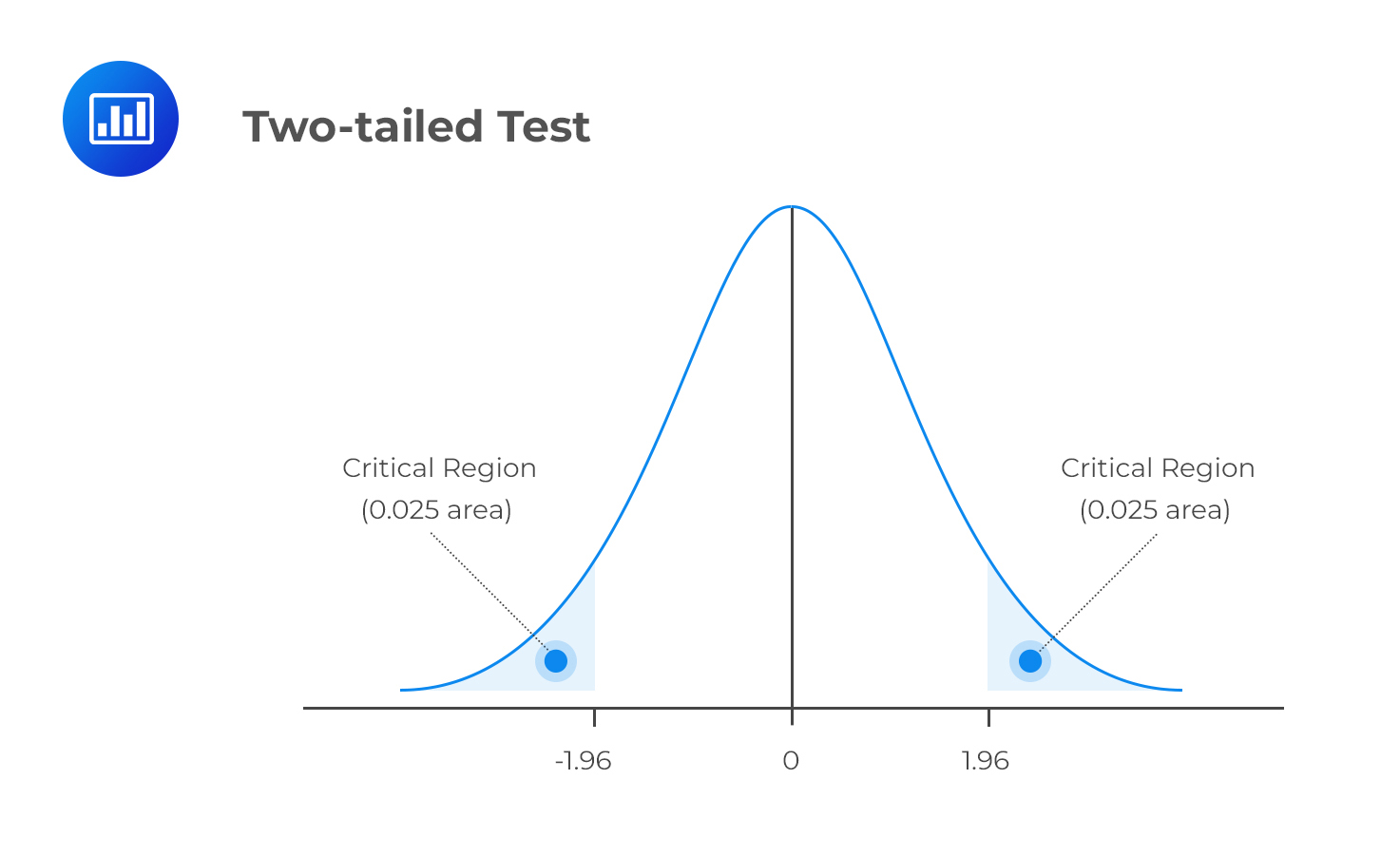# Estimated Regression Coefficients

The intercept term is defined as the value of the dependent variable when the independent variables are zero. On the other hand, each slope coefficient is the estimated change in the value of the dependent variable for a one-unit change in the value of the respective independent variable, keeping the other independent variables constant. Slope coefficients are also called partial slope coefficients.

### Example: Understanding Slop Coefficients

Consider the multiple regression of the price of USDX on inflation rates and real rates of interest:

$$P=81-276INF+902IR$$

Where:

• P = Price of the US Dollar index.
• INF = Annual inflation rate.
• IR = Annual real rate of interest.

The regression equation is interpreted as follows:

The intercept term of 81 implies that the price of USDX is $81 when both the inflation rate and real interest rate are 0. A 1% increase in the inflation rate leads to a$276 decrease in the price of USDX, keeping real interest rates constant. On the other hand, a 1% increase in the real interest rate leads to a \$902 increase in the price of USDX, keeping the inflation rate constant.

## Interpreting p-values

A p-value is the smallest significance level $$(\alpha)$$ at which we reject the null hypothesis $$(H_{0})$$. In other words, a p-value quickly allows us to establish if an independent variable is significant at a significance level such as 5%.

A p-value below 0.05 indicates a significant result. The null hypothesis $$H_0$$ should be rejected when the p-value $$< 0.05$$. The following table illustrates two-tailed p-values, which is what we will be concentrating on throughout these readings (except for F-tests, which are one-sided tests).

###Example: Interpreting p-values

Consider the following multiple regression results of the price of USDX versus inflation rate and real interest rate.

$$\small{\begin{array}{l|c|c|c|c}{}& \textbf{Coefficients} & \textbf{Standard Error} & \textbf{t-statistic} & \textbf{p-value}\\ \hline\text{Intercept} & 81 & 7.9659 & 10.1296 & 0.0000\\ \hline\text{Inflation rates} & -276 & 233.0748 & -1.1833 & 0.2753\\ \hline\text{Real interest rates} & 902 & 279.6949 & 3.2266 & 0.0145\\ \end{array}}$$

The above results imply that the real interest rate is statistically significant at the 5% level. This is because the p-value, 0.0145, is less than 0.05.

On the other hand, real inflation rate is not significant. This can be seen be corroborated by the high standard error, low t-statistic, and relatively high p-value above 0.05.

## Question

Adil Suleman, CFA, wishes to establish the possible drivers of a company’s percentage return on capital (ROC). Suleman identifies performance measures such as the profit margin (%), sales, and debt ratio as possible drivers of ROC. He obtains the following results from the regression of ROC on profit margin (%), sales, and the debt ratio.

$$\textbf{Exhibit 1: Summary Output}$$

$$\small{\begin{array}{|l|l|l|l|l|l|}\hline{}& \textbf{Coefficients} & \textbf{Standard Error} & \textbf{t-statistic} & \textbf{p-value} \\ \hline\text{Intercept} & 8.6531 & 0.9174 & 9.4323 & 0.0000 \\ \hline \text{Sales} & 0.0009 & 0.0005 & 1.7644 & 0.0922\\ \hline\text{Debt ratio} & 0.0229 & 0.0165 & 1.3880 & 0.1797 \\ \hline\text{Profit margin (%)} & 0.2996 & 0.0564 & 5.3146 & 0.0000\\ \hline\end{array}}$$

Which of the independent variables is (are) most likely statistically significantly different from zero at the 5% significance level, assuming the sample size is 25?

A. Profit margin.

B. Sales and profit margin.

C. Sales and debt ratio.

### Solution

An independent variable is statistically significant if its p-value is less than the significance level, in this case, 5%, or 0.05. Therefore, only the profit margin is statistically significantly different from zero at the 5% significance level.

LOS 2 (b) Interpret estimated regression coefficients and their p-value.

Shop CFA® Exam Prep

Offered by AnalystPrepLevel I
Level II
Level III
All Three Levels
Featured Shop FRM® Exam PrepFRM Part I
FRM Part II
FRM Part I & Part II
Learn with Us

Subscribe to our newsletter and keep up with the latest and greatest tips for success
Shop Actuarial Exams PrepExam P (Probability)
Exam FM (Financial Mathematics)
Exams P & FMGMAT® Complete CourseDaniel Glyn
2021-03-24
I have finished my FRM1 thanks to AnalystPrep. And now using AnalystPrep for my FRM2 preparation. Professor Forjan is brilliant. He gives such good explanations and analogies. And more than anything makes learning fun. A big thank you to Analystprep and Professor Forjan. 5 stars all the way!michael walshe
2021-03-18
Professor James' videos are excellent for understanding the underlying theories behind financial engineering / financial analysis. The AnalystPrep videos were better than any of the others that I searched through on YouTube for providing a clear explanation of some concepts, such as Portfolio theory, CAPM, and Arbitrage Pricing theory. Watching these cleared up many of the unclarities I had in my head. Highly recommended.Nyka Smith
2021-02-18
Every concept is very well explained by Nilay Arun. kudos to you man!2021-02-13